# Precalculus : Solve a Quadratic Equation

## Example Questions

### Example Question #1 : Solve A Quadratic Equation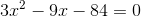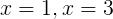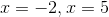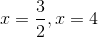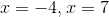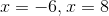Explanation:

When solving a quadratic equation, the first thing to look for is whether or not it can be factored, as this is most often the easiest and fastest method if the quadratic can in fact be factored. We can see that each of the terms in the given equation have a common factor of 3, so it will be easier to factor the quadratic if we first factor out the 3: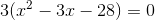Now we're left with a polynomial where we need to find two numbers whose product is -28 and whose sum is -3. Thinking about the factors of 28, we can see that 4 and 7 will yield -3 if 7 is negative and 4 is positive, so we now have our factorization: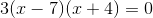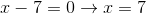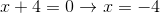### Example Question #2 : Solve A Quadratic Equation

Find the root(s) of the following quadratic equation?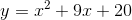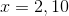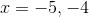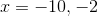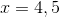Explanation:

To find the roots of an equation in the form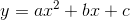, you use the quadratic formula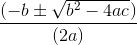.

In our case, we have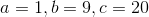This gives us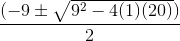which simplifies to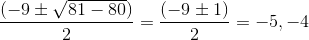### Example Question #3 : Solve A Quadratic Equation

Given the function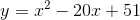, find a possible root for this quadratic.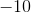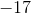Explanation:

Factorizeand set this equation equal to zero.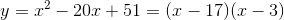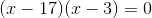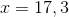The answeris one of the possible choices.

### Example Question #4 : Solve A Quadratic Equation

Solve the quadratic equation for.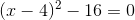Explanation:

There are two solutions;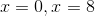.

We proceed as follows.Addto both sides.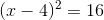Take the square root of both sides, remember to introduce plus/minus on the right side since you are introducing a square root into your work.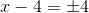Addto both sides.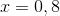### Example Question #5 : Solve A Quadratic Equation

Solve the quadratic equation for.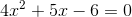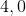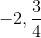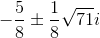Explanation:

For any quadtratic equation of the form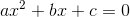, the quadratic formula is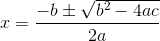Plugging in our given values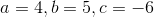we have: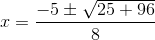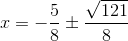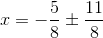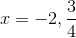### Example Question #6 : Solve A Quadratic Equation

Find the roots of the equation.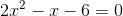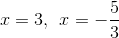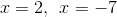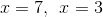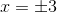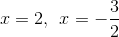Explanation:

Use either the quadratic formula or factoring to solve the quadratic equation.

Using factoring, we want to find which factors of six when multiplied with the factors of two and then added together result in negative one.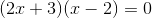let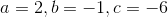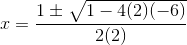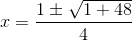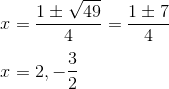### Example Question #7 : Solve A Quadratic Equation

Solve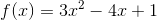.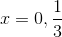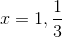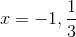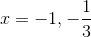To solve this equation, use trial and error to factor it. Since the leading coefficient is, there is only one way to get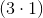, so that is helpful reminder. Once it's properly factored, you get: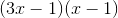. Then, set both of those expressions equal toto get your roots:.